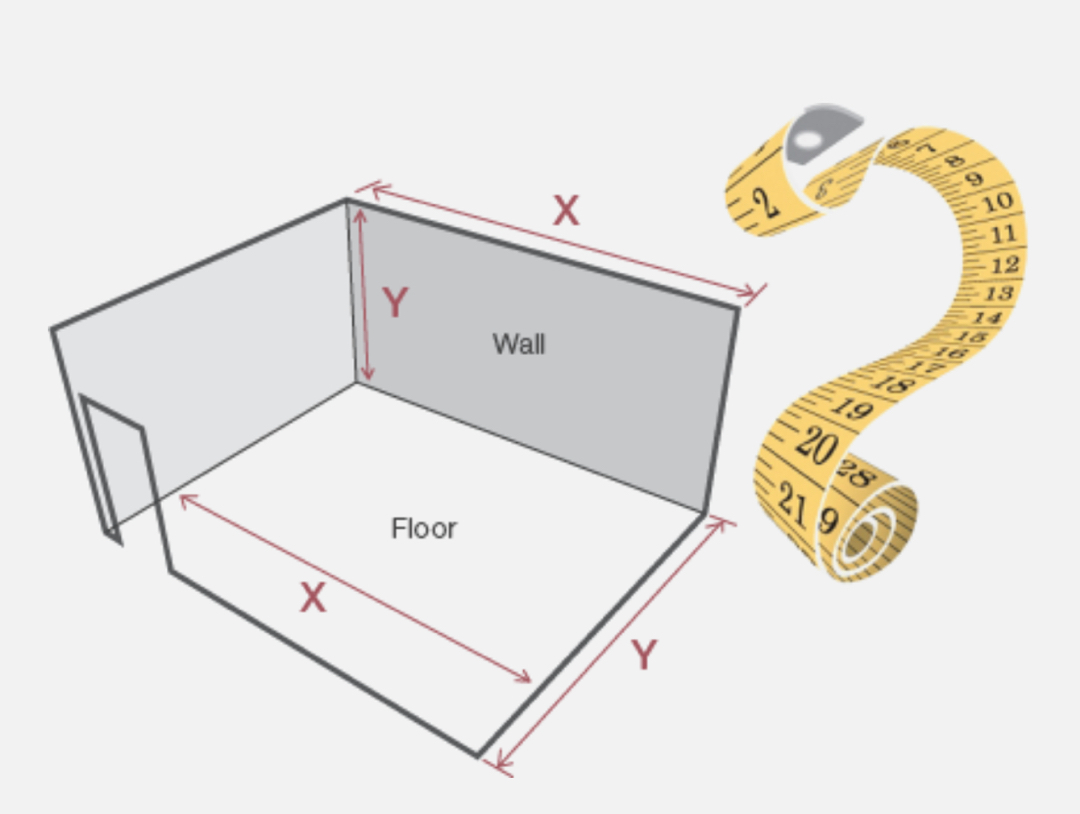How to measure upSimply measure each wall or floor and multiply the width by the depth (for floors) or width by the height you want to tile up to (for walls) to give the square metre area. If you are tiling more than one wall repeat for each wall and add the measurements together to find your total area.

If there are large obstructions in the wall such as doors and windows, multiply the width by the height of the obstruction then subtract that from the total area you need to tile.

We recommend adding at least 10% to your total area to allow for wastage when tiles have to be cut at the edges of the room.

Calculate how many of these tiles you will need

Enter the dimensions of your room (or the square metre area if you’ve already worked it out) and the size of your preferred tile and let our handy tile calculator do the hard work for you.

Enter tile dimensions (cm)
Enter your wall or floor dimensions (m)